Courses

# Test: Overlapping SETS- 2

## 10 Questions MCQ Test Quantitative Aptitude for GMAT | Test: Overlapping SETS- 2

Description
This mock test of Test: Overlapping SETS- 2 for GMAT helps you for every GMAT entrance exam. This contains 10 Multiple Choice Questions for GMAT Test: Overlapping SETS- 2 (mcq) to study with solutions a complete question bank. The solved questions answers in this Test: Overlapping SETS- 2 quiz give you a good mix of easy questions and tough questions. GMAT students definitely take this Test: Overlapping SETS- 2 exercise for a better result in the exam. You can find other Test: Overlapping SETS- 2 extra questions, long questions & short questions for GMAT on EduRev as well by searching above.
QUESTION: 1

### What percent of the students at Jefferson High School study French but not Spanish? (1) 30% of all students at Jefferson High School study French. (2) 40% of all students at Jefferson High School do not study Spanish.

Solution:

This is an overlapping sets problem. This question can be effectively solved with a double-set matrix composed of two overlapping sets: [Spanish/Not Spanish] and [French/Not French]. When constructing a double-set matrix, remember that the two categories adjacent to each other must be mutually exclusive, i.e. [French/not French] are mutually exclusive, but [French/not Spanish] are not mutually exclusive. Following these rules, let’s construct and fill in a double-set matrix for each statement. To simplify our work with percentages, we will also pick 100 for the total number of students at Jefferson High School.
INSUFFICIENT: While we know the percentage of students who take French and, from that information, the percentage of students who do not take French, we do not know anything about the students taking Spanish. Therefore we don't know the percentage of students who study French but not Spanish, i.e. the number in the target cell denoted with x.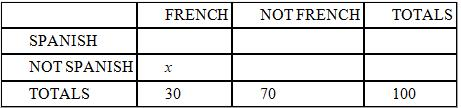(2) INSUFFICIENT: While we know the percentage of students who do not take Spanish and, from that information, the percentage of students who do take Spanish, we do not know anything about the students taking French. Therefore we don't know the percentage of students who study French but not Spanish, i.e. the number in the target cell denoted with x.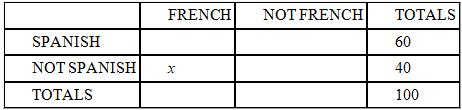AND (2) INSUFFICIENT: Even after we combine the two statements, we do not have sufficient information to find the percentage of students who study French but not Spanish, i.e. to fill in the target cell denoted with x.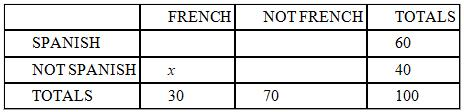QUESTION: 2

### If none of the students are ambidextrous, what percentage of the 20 students in Mr. Henderson's class are left-handed? (1) Of the 12 girls in the class, 25% are left-handed. (2) 5 of the boys in the class are right-handed.

Solution:

For this overlapping sets problem, we want to set up a double-set matrix.  The first set is boys vs. girls; the second set is left-handers vs. right-handers.

The only number currently in our chart is that given in the question: 20, the total number of students.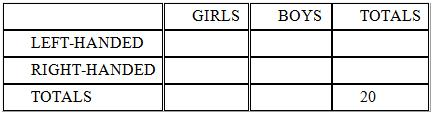INSUFFICIENT:  We can figure out that three girls are left-handed, but we know nothing about the boys.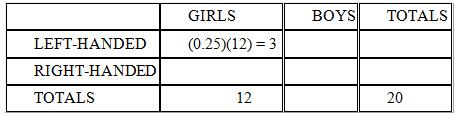(2) INSUFFICIENT:  We can't figure out the number of left-handed boys, and we know nothing about the girls.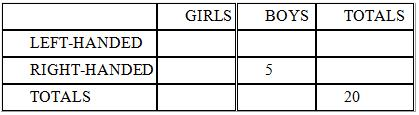(1) AND (2) SUFFICIENT:  If we combine both statements, we can get the missing pieces we need to solve the problem. Since we have 12 girls, we know that there are 8 boys.  If five of them are right-handed, then three of them must be left-handed.  Add that to the three left-handed girls, and we know that a total of 6 students are left-handed.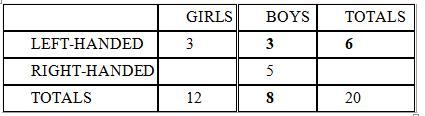QUESTION: 3

### Guests at a recent party ate a total of fifteen hamburgers. Each guest who was neither a student nor a vegetarian ate exactly one hamburger. No hamburger was eaten by any guest who was a student, a vegetarian, or both. If half of the guests were vegetarians, how many guests attended the party? (1) The vegetarians attended the party at a rate of 2 students to every 3 non-students, half the rate for non-vegetarians. (2) 30% of the guests were vegetarian non-students.

Solution:

For this overlapping set problem, we want to set up a two-set table to test our possibilities.  Our first set is vegetarians vs. non-vegetarians; our second set is students vs. non-students.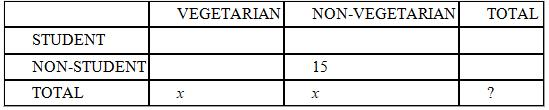We are told that each non-vegetarian non-student ate exactly one of the 15 hamburgers, and that nobody else ate any of the 15 hamburgers. This means that there were exactly 15 people in the non-vegetarian non-student category. We are also told that the total number of vegetarians was equal to the total number of non-vegetarians; we represent this by putting the same variable in both boxes of the chart.
The question is asking us how many people attended the party; in other words, we are being asked for the number that belongs in the bottom-right box, where we have placed a question mark.
The second statement is easier than the first statement, so we'll start with statement (2).
(2) INSUFFICIENT: This statement gives us information only about the cell labeled "vegetarian non-student"; further it only tells us the number of these guests as a percentage of the total guests.  The 30% figure does not allow us to calculate the actual number of any of the categories.
SUFFICIENT: This statement provides two pieces of information.  First, the vegetarians attended at the rate, or in the ratio, of 2:3 students to non-students.  We're also told that this 2:3 rate is half the rate for non-vegetarians.  In order to double a rate, we double the first number; the rate for non-vegetarians is 4:3  We can represent the actual numbers of non-vegetarians as 4a and 3a and add this to the chart below.  Since we know that there were 15 non-vegetarian non-students, we know the missing common multiple, a,  is 15/3 = 5.  Therefore, there were (4)(5) = 20 non-vegetarian students and 20 + 15 = 35 total non-vegetarians (see the chart below).  Since the same number of vegetarians and non-vegetarians attended the party, there were also 35 vegetarians, for a total of 70 guests.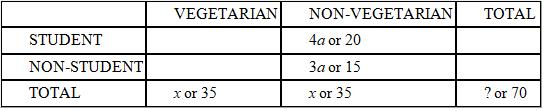QUESTION: 4

To receive a driver license, sixteen year-olds at Culliver High School have to pass both a written and a
practical driving test. Everyone has to take the tests, and no one failed both tests. If 30% of the 16 year-olds who passed the written test did not pass the practical, how many sixteen-year-olds at Culliver High School
(1) There are 188 sixteen year-olds at Culliver High School.
(2) 20% of the sixteen year-olds who passed the practical test failed the written test.

Solution:

For an overlapping set question, we can use a double-set matrix to organize the information and solve. The two sets in this question are the practical test (pass/fail) and the written test (pass/fail).

From the question we can fill in the matrix as follows. In a double-set matrix, the sum of the first two rows equals the third and the sum of the first two columns equals the third. The bolded value was derived from the other given values. The question asks us to find the value of .7x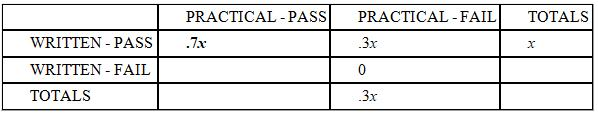(1) INSUFFICIENT: If we add the total number of students to the information from the question, we do not have enough to solve for .7x.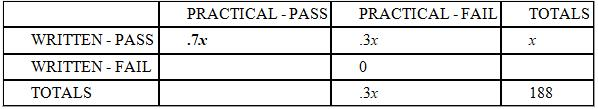(2) INSUFFICIENT: If we add the fact that 20% of the sixteen year-olds who passed the practical test failed the written test to the original matrix from the question, we can come up with the relationship .7x = .8y. However, that is not enough to solve for .7x.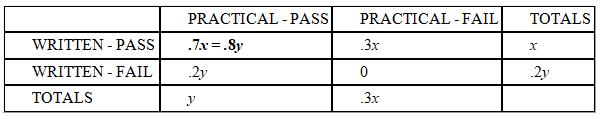(1) AND (2) SUFFICIENT: If we combine the two statements we get a matrix that can be used to form two relationships between x and y: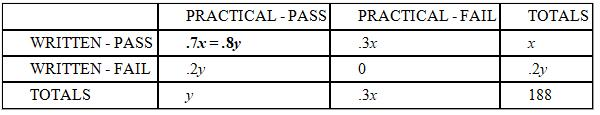.7x = .8y
y + .3x = 188

This would allow us to solve for x and in turn find the value of .7x, the number of sixteen year-olds who received a driver license.

QUESTION: 5

At a charity fundraiser, 180 of the guests had a house both in the Hamptons and in Palm Beach. If not
everyone at the fundraiser had a house in either the Hamptons or Palm Beach, what is the ratio of the
number of people who had a house in Palm Beach but not in the Hamptons to the number of people who had
a house in the Hamptons but not in Palm Beach?
(1) One-half of the guests had a house in Palm Beach.
(2) Two-thirds of the guests had a house in the Hamptons

Solution:

For an overlapping set problem we can use a double-set matrix to organize our information and solve. We are told in the question stem that 180 guests have a house in the Hamptons and a house in Palm Beach. We can insert this into our matrix as follows: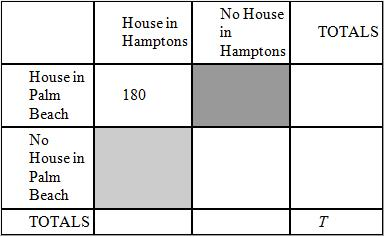The question is asking us for the ratio of the darkly shaded box to the lightly shaded box.

INSUFFICIENT: Since one-half of all the guests had a house in Palm Beach, we can fill in the matrix as follows: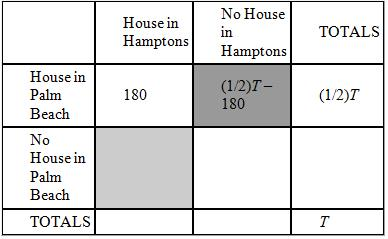We cannot find the ratio of the dark box to the light box from this information alone.

(2) INSUFFICIENT: Statement 2 tells us that two-thirds of all the guests had a house in the Hamptons. We can insert this into our matrix as follows: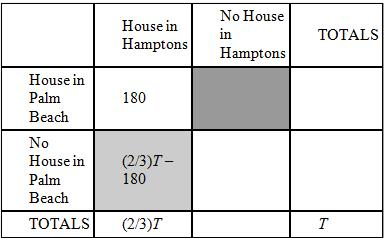We cannot find the ratio of the dark box to the light box from this information alone.

(1) AND (2) INSUFFICIENT:  we can fill in our matrix as follows.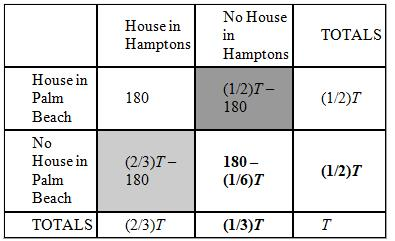The ratio of the number of people who had a house in Palm Beach but not in the Hamptons to the number of people who had a house in the Hamptons but not in Palm Beach (i.e. dark to light) will be: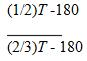This ratio doesn’t have a constant value; it depends on the value of T. We can try to solve for T by filling out the rest of the values in the matrix (see the bold entries above); however, any equation that we would build using these values reduces to a redundant statement of T = T. This means there isn’t enough unique information to solve for T.

QUESTION: 6

Recently Mary gave a birthday party for her daughter at which she served both chocolate and strawberry ice
cream. There were 8 boys who had chocolate ice cream, and nine girls who had strawberry. Everybody there
had some ice cream, but nobody tried both. What is the maximum possible number of girls who had some
chocolate ice cream?
Exactly thirty children attended the party.
Fewer than half the children had strawberry ice cream.

Solution:

Since there are two different classes into which we can divide the participants, we can solve this using a double-set matrix. The two classes into which we'll divide the participants are Boys/Girls along the top (as column labels), and Chocolate/Strawberry down the left (as row labels).

T
he problem gives us the following data to fill in the initial double-set matrix. We want to know if we can determine the maximum value of a, which represents the number of girls who ate chocolate ice cream.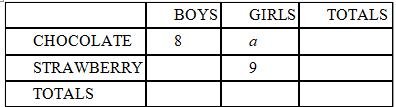(1) SUFFICIENT: Statement (1) tells us that exactly 30 children came to the party, so we'll fill in 30 for the grand total. Remember that we're trying to maximize a.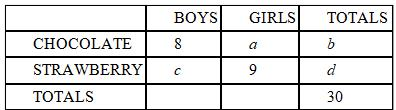In order to maximize a, we must maximize b, the total number of chocolate eaters. Since
b + d = 30, implying b = 30 - d, we must minimize d to maximize b. To minimize d we must minimize c. The minimum value for c is 0, since the question doesn't say that there were necessarily boys who had strawberry ice cream.

Now that we have an actual value for c, we can calculate forward to get the maximum possible value for a. If c = 0, since we know that c + 9 = d, then d = 9. Since b + d = 30, then b = 21. Given that 8 + a = b and b = 21, then a = 13, the maximum value we were looking for. Therefore statement (1) is sufficient to find the maximum number of girls who ate chocolate.

(2) INSUFFICIENT:  Knowing only that fewer than half of the people ate strawberry ice cream doesn't allow us to fill in any of the boxes with any concrete numbers.  Therefore statement (2) is insufficient.

QUESTION: 7

Many of the students at the International School speak French or German or both. Among the students who
speak French, four times as many speak German as don't. In addition, 1/6 of the students who don't speak
German do speak French. What fraction of the students speak German?
(1) Exactly 60 students speak French and German.
(2) Exactly 75 students speak neither French nor German.

Solution:

Since we are dealing with overlapping sets and there are two independent criteria, the best way to solve this problem is with a double-set matrix.
The first statement in the question stem tells us that of the students who speak French (represented by the first column), four times as many speak German as don't. This information yields the following entries in the double-set matrix: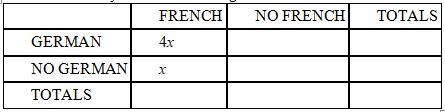The second statement in the question stem tells us that 1/6 of the of the students who don't speak German do speak French. This is fact represented in the double-set matrix as follows: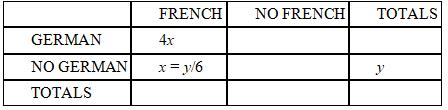Now since x = y/6, we can get rid of the new variable y and keep all the expressions in terms of x.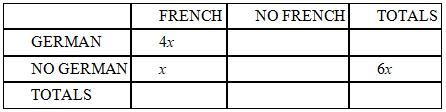Now we can fill in a few more boxes using the addition rules for the double-set matrix.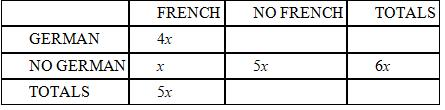The main question to be answered is what fraction of the students speak German, a fraction represented by A/B in the final double-set matrix. So, if statements (1) and/or (2) allow us to calculate a numerical value for A/B, we will be able to answer the question.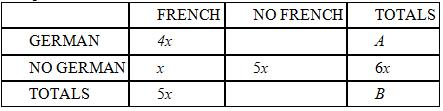(1) INSUFFICIENT: Statement (1) tells us that 60 students speak French and German, so 4x = 60 and x = 15. We can now calculate any box labeled with an x, but this is still insufficient to calculate A, B, or A/B.

(2) INSUFFICIENT: Statement (2) tells us that 75 students speak neither French nor German, so 5x = 75 and x = 15. Just as with Statement (1), we can now calculate any box labeled with an x, but this is still insufficient to calculate A, B, or A/B.

(1) AND (2) INSUFFICIENT: Since both statements give us the same information (namely, that x = 15), putting the two statements together does not tell us anything new. Therefore (1) and (2) together are insufficient.

QUESTION: 8

Each member of a pack of 55 wolves has either brown or blue eyes and either a white or a grey coat. If there
are more than 3 blue-eyed wolves with white coats, are there more blue-eyed wolves than brown-eyed
wolves?
(1) Among the blue-eyed wolves, the ratio of grey coats to white coats is 4 to 3.
(2) Among the brown-eyed wolves, the ratio of white coats to grey coats is 2 to 1.

Solution:

In an overlapping set problem, we can use a double set matrix to organize the information and solve.
From information given in the question, we can fill in the matrix as follows: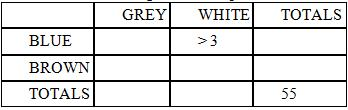The question is asking us if the total number of blue-eyed wolves (fourth column, second row) is greater than the total number of brown-eyed wolves (fourth column, third row).

(1) INSUFFICIENT. This statement allows us to fill in the matrix as below. We have no information about the total number of brown-eyed wolves.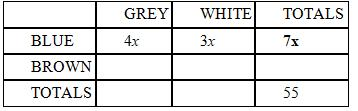(2) INSUFFICIENT. This statement allows us to fill in the matrix as below. We have no information about the total number of blue-eyed wolves.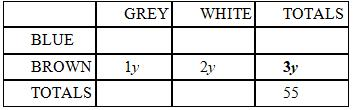TOGETHER, statements (1) + (2) are SUFFICIENT. Combining both statements, we can fill in the matrix as follows: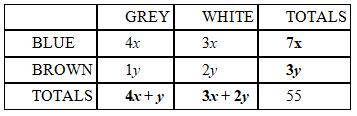Using the additive relationships in the matrix, we can derive the equation 7x + 3y = 55 (notice that adding the grey and white totals yields the same equation as adding the blue and brown totals).

The original question can be rephrased as "Is 7x > 3y?"

On the surface, there seems to NOT be enough information to solve this question. However, we must consider some of the restrictions that are placed on the values of x and y:

(1) x and y must be integers (we are talking about numbers of wolves here and looking at the table, y, 3x and 4x must be integers so x and y must be integers)

(2) x must be greater than 1 (the problem says there are more than 3 blue-eyed wolves with white coats so 3x must be greater than 3 or x > 1)

Since x and y must be integers, there are only a few x,y values that satisfy the equation 7x + 3y = 55. By trying all integer values for x from 1 to 7, we can see that the only possible x,y pairs are: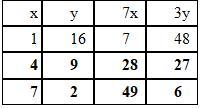Since x cannot be 1, the only two pairs yield 7x values that are greater than the corresponding 3y values (28 > 27 and 49 > 6).

QUESTION: 9

What percentage of the current fourth graders at Liberation Elementary School dressed in costume for
Halloween for the past two years in a row (both this year and last year)?
(1) 60% of the current fourth graders at Liberation Elementary School dressed in costume for Halloween this
year.
(2) Of the current fourth graders at Liberation Elementary School who did not dress in costume for
Halloween this year, 80% did not dress in costume last year.

Solution:

We can divide the current fourth graders into 4 categories:
The percentage that dressed in costume this year ONLY.
(2) The percentage that dressed in costume last year ONLY.
(3) The percentage that did NOT dress in costume either this year or last year.
(4) The percentage that dressed in costume BOTH years.

We need to determine the last category (category 4) in order to answer the question.
INSUFFICIENT:  Let's assume there are 100 current fourth graders (this simply helps to make this percentage question more concrete). 60 of them dressed in costume this year, while 40 did not. However, we don't know how many of these 60 dressed in costume last year, so we can't divide this 60 up into categories 1 and 2.
INSUFFICIENT:  This provides little relevant information on its own because we don't know how many of the students didn't dress up in costumes this year and the statement references that value.

(1) AND (2) INSUFFICIENT:  From statement 1 we know that 60 dressed up in costumes this year, but 40 did not. Statement 2 tells us that 80% of these 40, or 32, didn't dress up in costumes this year either.  This provides us with a value for category 3, from which we can derive a value for category 2 (8).  However, we still don't know how many of the 60 costume bearers from this year wore costumes last year.

Since this is an overlapping set problem, we could also have used a double-set matrix to organize our information and solve. Even with both statements together, we can not find the value for the Costume Last Year / Costume This Year cell.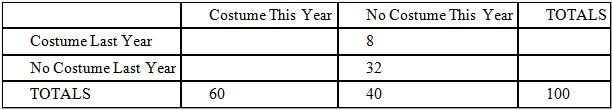QUESTION: 10

Of all the houses on Kermit Lane, 20 have front porches, 20 have front yards, and 40 have back yards. How
many houses are on Kermit Lane?
(1) No house on Kermit Lane is without a back yard.
(2) Each house on Kermit Lane that has a front porch does not have a front yard.

Solution: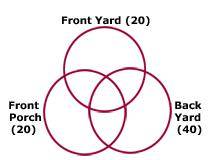A Venn-Diagram is useful to visualize this problem.
Notice that the Venn diagram allows us to see the 7 different types of houses on Kermit lane. Each part of the diagram represents one type of house. For example, the center section of the diagram represents the houses that contain all three amenities (front yard, front porch, and back yard). Keep in mind that there may also be some houses on Kermit Lane that have none of the 3 amenities and so these houses would be outside the diagram.
SUFFICIENT: This tells us that no house on Kermit Lane is without a backyard. Essentially this means that there are 0 houses in the three sections of the diagram that are NOT contained in the Back Yard circle. It also means that there are 0 houses outside of the diagram. Since we know that 40 houses on Kermit Lane contain a back yard, there must be exactly 40 houses on Kermit Lane.
INSUFFICIENT:  This tells us that each house on Kermit Lane that has a front porch does not have a front yard. This means that there are 0 houses in the two sections of the diagram in which Front Yard overlaps with Front Porch. However, this does not give us information about the other sections of the diagram. Statement (2) ALONE is not sufficient.